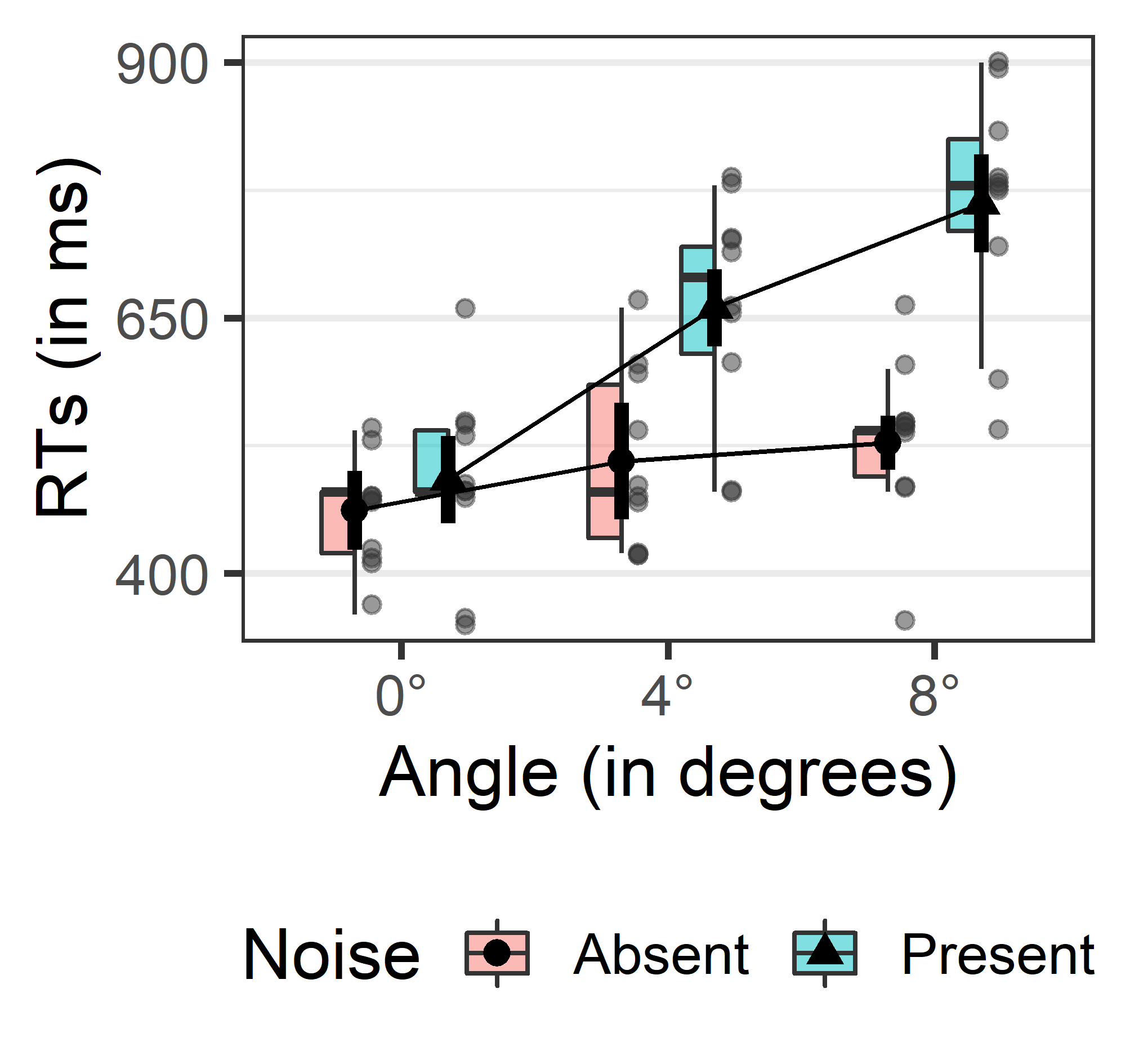Want to share your content on R-bloggers? click here if you have a blog, or here if you don't.

I am happy to announce that a new version of `afex` (version 0.22-1) has appeared on CRAN. This version comes with two major changes, for more see the NEWS file. To get the new version including all packages used in the examples run:

```install.packages("afex", dependencies = TRUE)
```

First, `afex` does not load or attach package `emmeans` automatically anymore. This reduces the package footprint and makes it more lightweight. If you want to use `afex` without using `emmeans`, you can do this now. The consequence of this is that you have to attach `emmeans` explicitly if you want to continue using `emmeans()` et al. in the same manner. Simply add `library("emmeans")` to the top of your script just below `library("afex")` and things remain unchanged. Alternatively, you can use `emmeans::emmeans()` without attaching the package.

Second and more importantly, I have added a new plotting function to `afex`. `afex_plot()` visualizes results from factorial experiments combining estimated marginal means and associated uncertainties (i.e., error bars) in the foreground with a depiction of the raw data in the background. Currently, `afex_plots()` supports ANOVAs and mixed models fitted with `afex` as well as mixed models fitted with `lme4` (support for more models will come in the next version). As shown in the example below, `afex_plots()` makes it easy to produce nice looking plots that are ready to be incorporated into publications. Importantly, `afex_plots()` allows different types of error bars, including within-subjects confidence intervals, which makes it particularly useful for fields where such designs are very common (e.g., psychology). Furthermore, `afex_plots()` is built on `ggplot2` and designed in a modular manner, making it easy to customize the plot to ones personal preferences.

`afex_plot()` requires the fitted model object as first argument and then has three arguments determining which factor or factors are displayed how:
`x` is necessary and specifies the factor(s) plotted on the x-axis
`trace` is optional and specifies the factor(s) plotted as separate lines (i.e., with each factor-level present at each x-axis tick)
`panel` is optional and specifies the factor(s) which separate the plot into different panels.

The further arguments make it easy to customize the plot in various ways. A comprehensive overview is provided in the new vignette, further details, specifically regarding the question of which type of error bars are supported, is given on its help page (which also has many more examples).

Let us look at an example. We take data from a 3 by 2 within-subject experiment that also features prominently in the vignette. Note that we plot within-subjects confidence intervals (by setting `error = "within"`) and then customize the plot quite a bit by changing the theme, using nicer labels, removing some y-axis ticks, adding colour, and using a customized `geom` (`geom_boxjitter` from the `ggpol` package) for displaying the data in the background.

```library("afex")
library("ggplot2")
data(md_12.1)
aw <- aov_ez("id", "rt", md_12.1, within = c("angle", "noise"))

afex_plot(aw, x = "angle", trace = "noise", error = "within",
mapping = c("shape", "fill"), dodge = 0.7,
data_geom = ggpol::geom_boxjitter,
data_arg = list(
width = 0.5,
jitter.width = 0,
jitter.height = 10,
outlier.intersect = TRUE),
point_arg = list(size = 2.5),
error_arg = list(size = 1.5, width = 0),
factor_levels = list(angle = c("0°", "4°", "8°"),
noise = c("Absent", "Present")),
legend_title = "Noise") +
labs(y = "RTs (in ms)", x = "Angle (in degrees)") +
scale_y_continuous(breaks=seq(400, 900, length.out = 3)) +
theme_bw(base_size = 15) +
theme(legend.position="bottom", panel.grid.major.x = element_blank())

ggsave("afex_plot.png", device = "png", dpi = 600,
width = 8.5, height = 8, units = "cm")
```In the plot, the black dots are the means and the thick black lines the 95% within-subject confidence intervals. The raw data is displayed in the background with a half box plot showing the median and upper and lower quartile as well as the raw data. The raw data is jittered on the y-axis to avoid perfect overlap.

One final thing to note. In the vignette on CRAN as well as the help page there is an error in the code. The name of the argument for changing the labels of the factor-levels is `factor_levels` and not `new_levels`. The vignette linked above and here uses the correct argument name. This is already corrected on github and will be corrected on CRAN with the next release.